Select Page

# MCQ Answers for CBSE 12 Science Maths Continuity and Differentiability in English

MCQ Answers for CBSE 12 Science Maths Continuity and Differentiability in English to enable students to get Answers in a narrative video format for the specific question.

Expert Teacher provides MCQ Answers for CBSE 12 Science Maths Continuity and Differentiability through Video Answers in English language. This video solution will be useful for students to understand how to write an answer in exam in order to score more marks. This teacher uses a narrative style for a question from Continuity and Differentiability not only to explain the proper method of answering question, but deriving right answer too.

Please find the question below and view the Answer in a narrative video format.

Question:

## Similar Questions from CBSE, 12th Science, Maths, Continuity and Differentiability

Question 1 : If, findin terms of y alone. (View Answer Video)

Question 2 : Differentiate the functionwith respect to x. (View Answer Video)

Question 3 : Using the fact thatand the differentiation, obtain the sum formula for cosines. (View Answer Video)

Question 4 : Differentiate w.r.t.x the function. (View Answer Video)

Question 5 : Findfor the function. (View Answer Video)

### Inverse Trigonometric Functions

Question 1 : Write the value of(View Answer Video)

Question 2 : Write the principal value of(View Answer Video)

Question 3 :is equal to :

Question 4 : Solve for(View Answer Video)

Question 5 : Iffind x. (View Answer Video)

### Probability

Question 1 : If E and F are two events such that,, Find P(not E and not F). (View Answer Video)

Question 2 : A couple has 2 children. Find the probability that both are boys, if it is known that the older child is boy. (View Answer Video)

Question 3 : Evaluate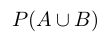, if 2P(A)=P(B)=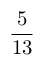and=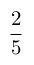. (View Answer Video)

Question 4 :  Probability of solving specific problem independently by A and B are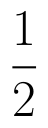and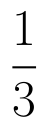respectively. If both try to solve the problem, independently, then find the probability that exactly one of them solves the problem.  (View Answer Video)

Question 5 : A die is thrown 6 times. If 'getting an odd number' is a 'success', what is the probability of obtaining:
Question 2 : The identity element for the binary operation * defined by a * b =, where a, b are the elements of a set of non-zero rational numbers, is, (View Answer Video)
Question 3 : Functionsare defined respectively, by, find(View Answer Video)
Question 5 : Ifis a relation on N, write the range of R. (View Answer Video)